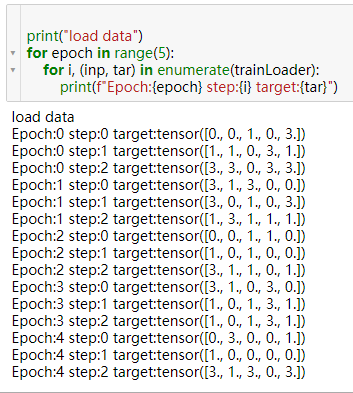# Is WeightedSampler really useful?

Because my dataset is imbalanced, I want to use torch.utils.data.WeightedSampler to sample data. Before do that I did a experiment, and the code is as follow:

``````import torch
from torch.utils.data.sampler import Sampler
from torch.utils.data import TensorDataset as dset

batch_size = 5
inputs = torch.randn(15,2)
# print(inputs)
target = torch.floor(4*torch.rand(15))
print(target)
trainData = dset(inputs, target)

count_labels = [sum(target==i) for i in range(4)]
print(count_labels)

num_sample = len(inputs)
weight = 1.0/torch.Tensor(count_labels).clone().detach()
print(weight)
sampler = torch.utils.data.sampler.WeightedRandomSampler(weight, num_sample)
trainLoader = torch.utils.data.DataLoader(trainData, batch_size , shuffle=False, sampler=sampler)
``````

The output is

``````tensor([0., 1., 3., 3., 2., 2., 0., 2., 1., 3., 2., 2., 2., 2., 3.])
[tensor(2, dtype=torch.uint8), tensor(2, dtype=torch.uint8), tensor(7, dtype=torch.uint8), tensor(4, dtype=torch.uint8)]
tensor([0.5000, 0.5000, 0.1429, 0.2500])
``````

Then I iterate load data in the following way:

``````print("load data")
for epoch in range(5):
for i, (inp, tar) in enumerate(trainLoader):
print(f"Epoch:{epoch} step:{i} target:{tar}")
``````

The result as follows:No matter how many times I tried, class 2 is never sampled. I wonder whether my code is wrong or the WeightedSampler is wrong?

The `weight` should provide a weight for each sample, while in your code it looks like you are trying to pass class weights.
Have a look at this example on how to use the `WeightedRandomSampler`.

3 Likes

Hi, ptrblck

In the code above, is there anything wrong about datatype conversion?
I just remove `.clone().detach()` on weight and add `.numpy()` to weight then pass to sampler, it works fine.
And the Pytorch raise a UserWarning when I run the snippet above:

``````UserWarning: To copy construct from a tensor, it is recommended to use sourceTensor.clone().detach() or sourceTensor.clone().detach().requires_grad_(True), rather than torch.tensor(sourceTensor).
``````

Does `WeightedRandomSampler` works only fine with `numpy array`?

1 Like

This warning seems to be related to this issue and should be fixed in the current master (and nightly builds).1 Like

That’s very kind.

What I am confused about is should we pass a `numpy.array` to the `WeightRandomSampler`, since in the snippet above it pass a sequence which wrap tensor to the sampler and it didn’t work.

In the code snipper, `weight` should be a `tensor` and should work fine.
Oh, thank you very much, it was my problem misunderstanding about the `weight` you have mentioned in your above post.